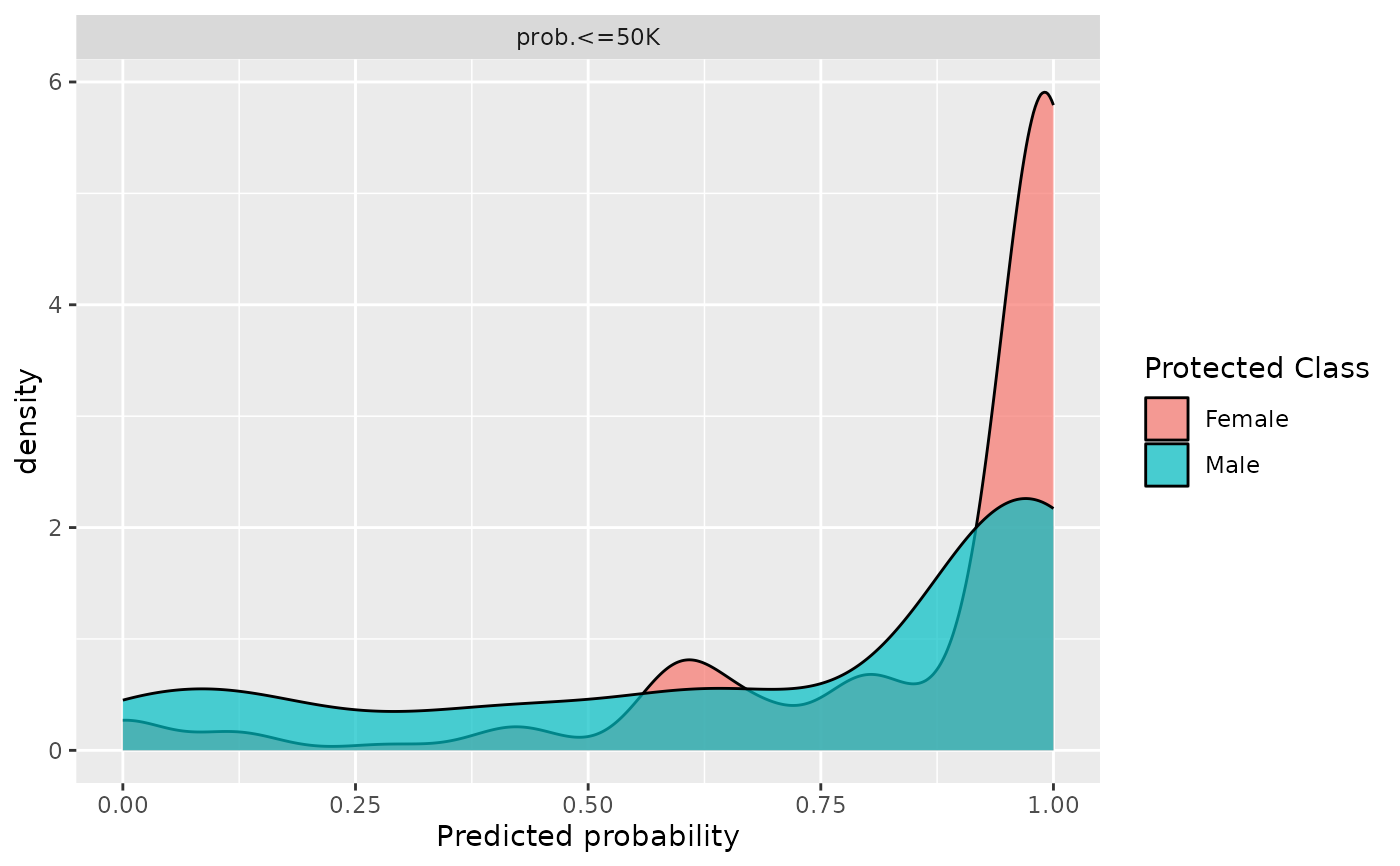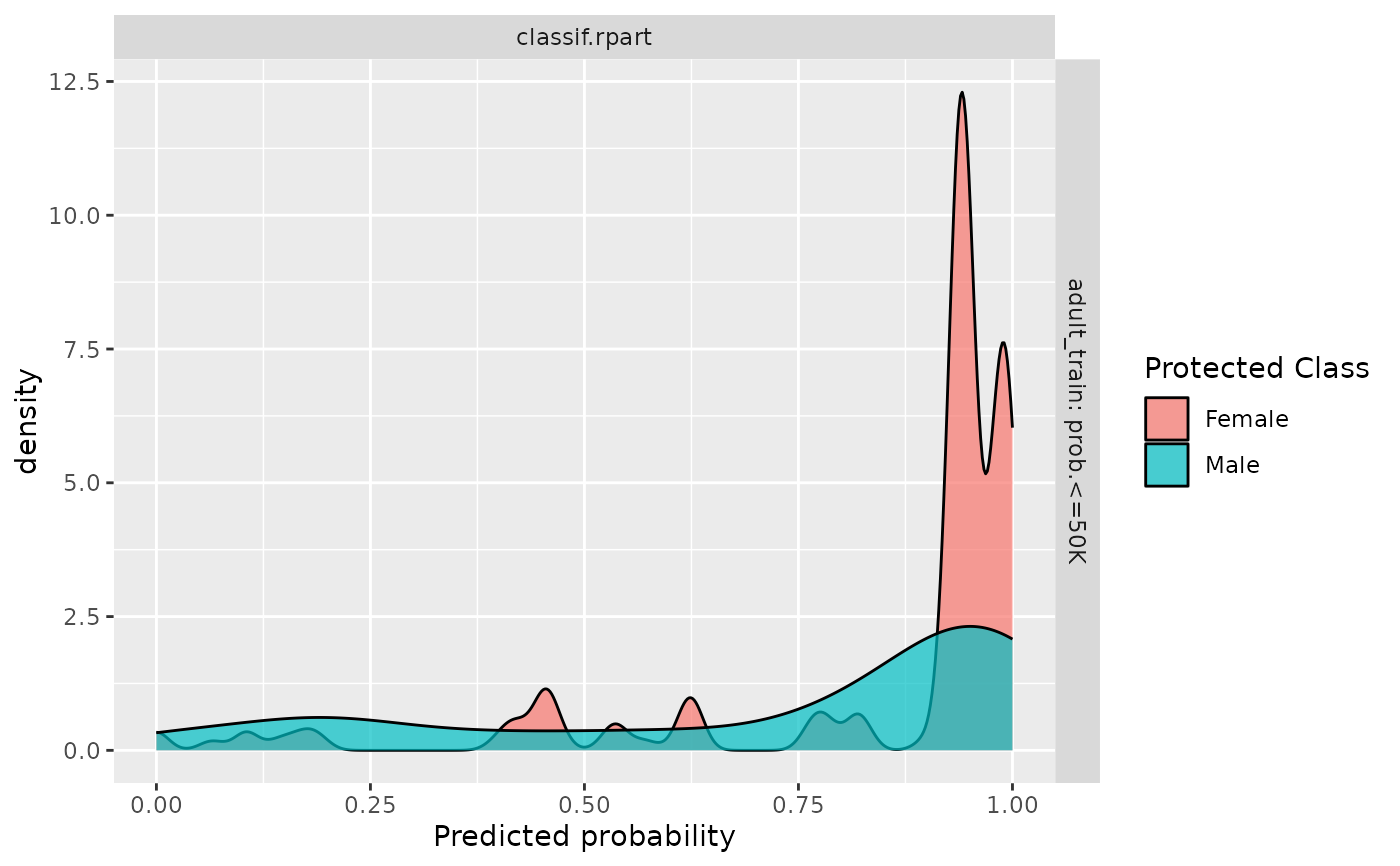Visualizes per-subgroup densities across learners, task and class. The plot is a combination of boxplot and violin plot. The y-axis shows the levels in protected columns. And the x-axis shows the predicted probability. The title for the plot will demonstrate which class for predicted probability.

## Usage

fairness_prediction_density(object, ...)

## Arguments

object

(PredictionClassif | ResampleResult | BenchmarkResult)
The binary class prediction object that will be evaluated. If PredictionClassif, a Task is required.

...

The arguments to be passed to methods, such as:

• task (TaskClassif)
The data task that contains the protected column.

• type character
The plot type. Either violin or density.

## Value

A 'ggplot2' object.

## Examples

library(mlr3learners)

task = tsk("adult_train")$filter(1:500) learner = lrn("classif.rpart", predict_type = "prob", cp = 0.001) learner$train(task)

# For prediction